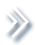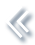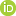####ArchivesReview Articles

## A class of admissible estimators of multiple regression coefficient with an unknown variance

Chengyuan Song ,

a Key Laboratory of Advanced Theory and Application in Statistics and Data Science-MOE, School of Statistics, East China Normal University, Shanghai, People's Republic of Chinasongchengyuanchina@163.com

Dongchu Sun

a Key Laboratory of Advanced Theory and Application in Statistics and Data Science-MOE, School of Statistics, East China Normal University, Shanghai, People's Republic of China;b Department of Statistics, University of Nebraska-Lincoln, Lincoln, NE, USAPages 190-201 | Received 10 Jun. 2019, Accepted 21 Jul. 2019, Published online: 20 Aug. 2019,
• Abstract
• References
• Citations

Abstract

Suppose that we observe y∣θ, τ∼Np(Xθ,τ−1Ip), where θ is an unknown vector with unknown precision τ. Estimating the regression coefficient θ with known τ has been well studied. However, statistical properties such as admissibility in estimating θ with unknown τ are not well studied. Han [(2009). Topics in shrinkage estimation and in causal inference (PhD thesis). Warton School, University of Pennsylvania] appears to be the first to consider the problem, developing sufficient conditions for the admissibility of estimating means of multivariate normal distributions with unknown variance. We generalise the sufficient conditions for admissibility and apply these results to the normal linear regression model. 2-level and 3-level hierarchical models with unknown precision τ are investigated when a standard class of hierarchical priors leads to admissible estimators of θ under the normalised squared error loss. One reason to consider this problem is the importance of admissibility in the hierarchical prior selection, and we expect that our study could be helpful in providing some reference for choosing hierarchical priors.

## References

1. Bayarri, M. J., Berger, J. O., Forte, A., & Garcła-Donato, G. (2012). Criteria for Bayesian model choice with application to variable selection. The Annals of Statistics40, 1550–1577. doi: 10.1214/12-AOS1013 [Crossref][Web of Science ®], [Google Scholar]
2. Berger, J. O. (1980). A robust generalized Bayes estimator and confidence region for a multivariate normal mean. The Annals of Statistics8, 716–761. doi: 10.1214/aos/1176345068 [Crossref][Web of Science ®], [Google Scholar]
3. Berger, J. O. (1985). Statistical decision theory and Bayesian analysis (2nd ed.). New York: Springer-Verlag Inc. [Crossref], [Google Scholar]
4. Berger, J. O., Pericchi, L. R., & Varshavsky, J. A. (1998). Bayes factors and marginal distributions in invariant situations. Sankhya, Series A, Indian Journal of Statistics60, 307–321. [Google Scholar]
5. Berger, J. O., & Strawderman, W. E. (1996). Choice of hierarchical priors: Admissibility in estimation of normal means. The Annals of Statistics24, 931–951. doi: 10.1214/aos/1032526950 [Crossref][Web of Science ®], [Google Scholar]
6. Berger, J. O., Strawderman, W., & Tang, D. (2005). Posterior propriety and admissibility of hyperpriors in normal hierarchical models. The Annals of Statistics33, 606–646. doi: 10.1214/009053605000000075 [Crossref][Web of Science ®], [Google Scholar]
7. Berger, J. O., Sun, D., & Song, C. (2018). An objective prior for hyperparameters in normal hierarchical models. Submitted. [Google Scholar]
8. Blyth, C. (1951). On minimax statistical decision procedures and their admissibility. The Annals of Mathematical Statistics22, 22–42. doi: 10.1214/aoms/1177729690 [Crossref], [Google Scholar]
9. Brown, L. D. (1971). Admissible estimators, recurrent diffusions, and insoluble boundary value problems. The Annals of Mathematical Statistics42, 855–903. doi: 10.1214/aoms/1177693318 [Crossref], [Google Scholar]
10. Cui, W., & George, E. I. (2008). Empirical Bayes vs. fully Bayes variable selection. Journal of Statistical Planning and Inference138, 888–900. doi: 10.1016/j.jspi.2007.02.011 [Crossref][Web of Science ®], [Google Scholar]
11. Eaton, M. L. (1989). Group invariance applications in statistics. Institute of Mathematical Statistics1, 1–133. [Google Scholar]
12. Fernández, C., Ley, E., & Steel, M. F. J. (2001). Benchmark priors for Bayesian model averaging. Journal of Econometrics100, 381–427. doi: 10.1016/S0304-4076(00)00076-2 [Crossref][Web of Science ®], [Google Scholar]
13. Foster, D. P., & George, E. I. (1994). The risk inflation criterion for multiple regression. The Annals of Statistics22, 1947–1975. doi: 10.1214/aos/1176325766 [Crossref][Web of Science ®], [Google Scholar]
14. Fraisse, A., Raoult, J., Robert, C., & Roy, M. (1990). Une condition nécessaire d'admissibilité et ses conséquences sur les estimateurs a˙a˙ rétrécisseur de la moyenne d'un vecteur normal. Canadian Journal of Statistics18, 213–220. doi: 10.2307/3315452 [Crossref], [Google Scholar]
15. George, E. I., & Foster, D. P. (2000). Calibration and empirical Bayes variable selection. Biometrika87, 731–747. doi: 10.1093/biomet/87.4.731 [Crossref][Web of Science ®], [Google Scholar]
16. Han, X. (2009). Topics in shrinkage estimation and in causal inference (PhD thesis). Warton School, University of Pennsylvania. [Google Scholar]
17. James, W., & Stein, C. (1961). Estimation with quadratic loss.Proceedings Fourth Berkeley Symposium in Mathematical Statistics and Probability (Vol. 1, pp. 361–3679). [Google Scholar]
18. Judge, G., Yancey, T., & Bock, M. (1983). Pre-test estimation under squared error loss. Economics Letters11, 347–352. doi: 10.1016/0165-1765(83)90028-9 [Crossref][Web of Science ®], [Google Scholar]
19. Kass, R. E., & Wasserman, L. (1995). A reference Bayesian test for nested hypotheses and its relationship to the Schwarz criterion. Journal of the American Statistical Association90, 928–934. doi: 10.1080/01621459.1995.10476592 [Taylor & Francis Online][Web of Science ®], [Google Scholar]
20. Katz, V. J. (2005). The history of Stokes' theorem. Mathematics Magazine52, 146–156. doi: 10.1080/0025570X.1979.11976770 [Taylor & Francis Online], [Google Scholar]
21. Liang, F., Paulo, R., Molina, G., Clyde, M., & Berger, J. O. (2008). Mixtures of g priors for Bayesian variable selection. Journal of the American Statistical Association103, 410–423. doi: 10.1198/016214507000001337 [Taylor & Francis Online][Web of Science ®], [Google Scholar]
22. Maruyama, Y., & Strawderman, W. (2005). A new class of generalized Bayes minimax ridge regression estimators. The Annals of Statistics33, 1753–1770. doi: 10.1214/009053605000000327 [Crossref][Web of Science ®], [Google Scholar]
23. Robert, C. (2007). The Bayesian choice: From decision-theoretic foundations to computational implementation. New York: Springer. [Google Scholar]
24. Stein, C. (1981). Estimation of the mean of a multivariate normal distribution. The Annals of Statistics9, 1135–1151. doi: 10.1214/aos/1176345632 [Crossref][Web of Science ®], [Google Scholar]
25. Strawderman, W. (1971). Proper Bayes minimax estimators of the multivariate normal mean. The Annals of Mathematical Statistics42, 385–388. doi: 10.1214/aoms/1177693528 [Crossref], [Google Scholar]
26. Strawderman, W. E. (1973). Proper Bayes minimax estimators of the multivariate normal mean vector for the case of common unknown variances. The Annals of Statistics1, 1189–1194. doi: 10.1214/aos/1176342567 [Crossref][Web of Science ®], [Google Scholar]
27. Willing, R., & Zhou, G. (2008). Generalized Bayes minimax estimators of the mean of multivariate normal distribution with unknown variance. Journal of Multivariate Analysis99, 2208–2220. doi: 10.1016/j.jmva.2008.02.016 [Crossref][Web of Science ®], [Google Scholar]
28. Zellner, A. (1986). On assessing prior distributions and Bayesian regression analysis with g-prior distributions. In: Goel, P. and Zellner, A., Eds., Bayesian Inference and Decision Techniques: Essays in Honor of Bruno de Finetti (pp. 233–243). New York: Elsevier Science Publishers, Inc. [Google Scholar]
29. Zellner, A., & Siow, A. (1980). Posterior odds ratios for selected regression hypotheses. In J. M. Bernardo, M. H.DeGroot, D. V. Lindley, & A. F. M. Smith (Eds.), Bayesian statistics (pp. 585–603). Valencia: University Press. [Google Scholar]